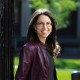# Doctrine of the (measurement) mean

Don’t invite me to dinner the night before an academic year begins.

You’ll find me in an armchair or sitting on my bed, laptop on my lap, journaling. I initiated the tradition the night before beginning college. I take stock of the past year, my present state, and hopes for the coming year.

Much of the exercise fosters what my high-school physics teacher called “an attitude of gratitude”: I reflect on cities I’ve visited, projects firing me up, family events attended, and subfields sampled. Other paragraphs, I want off my chest: Have I pushed this collaborator too hard or that project too little? Miscommunicated or misunderstood? Strayed too far into heuristics or into mathematical formalisms?

If only the “too much” errors, I end up thinking, could cancel the “too little.”

In one quantum-information context, they can.Imagine that you’ve fabricated the material that will topple steel and graphene; let’s call it a supermetatopoconsulator. How, you wonder, do charge, energy, and particles move through this material? You’ll learn by measuring correlators.

A correlator signals how much, if you poke this piece here, that piece there responds. At least, a two-point correlator does:$\langle A(0) B(\tau) \rangle$.$A(0)$ represents the poke, which occurs at time$t = 0$.$B(\tau)$ represents the observable measured there at$t = \tau$. The$\langle . \rangle$ encapsulates which state$\rho$ the system started in.

Condensed-matter, quantum-optics, and particle experimentalists have measured two-point correlators for years. But consider the three-point correlator$\langle A(0) B(\tau) C (\tau' ) \rangle$, or a$k$-point$\langle \underbrace{ A(0) \ldots M (\tau^{(k)}) }_k \rangle$, for any$k \geq 2$. Higher-point correlators relate more-complicated relationships amongst events. Four-pointcorrelators associated with multiple times signal quantum chaos and information scrambling. Quantum information scrambles upon spreading across a system through many-body entanglement. Could you measure arbitrary-point, arbitrary-time correlators?Supermetatopoconsulator (artist’s conception)

Yes, collaborators and I have written, using weak measurements. Weak measurements barely disturb the system being measured. But they extract little information about the measured system. So, to measure a correlator, you’d have to perform many trials. Moreover, your postdocs and students might have little experience with weak measurements. They might not want to learn the techniques required, to recalibrate their detectors, etc. Could you measure these correlators easily?

Yes, if the material consists of qubits,2 according to a paper I published with Justin Dressel, José Raúl González Alsonso, and Mordecai Waegell this summer. You could build such a system from, e.g., superconducting circuits, trapped ions, or quantum dots.

You can measure$\langle \underbrace{ A(0) B (\tau') C (\tau'') \ldots M (\tau^{(k)}) }_k \rangle$, we show, by measuring$A$ at$t = 0$, waiting until$t = \tau'$, measuring$B$, and so on until measuring$M$ at$t = \tau^{(k)}$. The$t$-values needn’t increase sequentially:$\tau''$ could be less than$\tau'$, for instance. You’d have to effectively reverse the flow of time experienced by the qubits. Experimentalists can do so by, for example, flipping magnetic fields upside-down.

Each measurement requires an ancilla, or helper qubit. The ancilla acts as a detector that records the measurement’s outcome. Suppose that$A$ is an observable of qubit #1 of the system of interest. You bring an ancilla to qubit 1, entangle the qubits (force them to interact), and look at the ancilla. (Experts: You perform a controlled rotation on the ancilla, conditioning on the system qubit.)

Each trial yields$k$ measurement outcomes. They form a sequence$S$, such as$(1, 1, 1, -1, -1, \ldots)$. You should compute a number$\alpha$, according to a formula we provide, from each measurement outcome and from the measurement’s settings. These numbers form a new sequence$S' = \mathbf{(} \alpha_S(1), \alpha_S(1), \ldots \mathbf{)}$. Why bother? So that you can force errors to cancel.

Multiply the$\alpha$’s together,$\alpha_S(1) \times \alpha_S(1) \times \ldots$, and average the product over the possible sequences$S$. This average equals the correlator$\langle \underbrace{ A(0) \ldots M (\tau^{(k)}) }_k \rangle$. Congratulations; you’ve characterized transport in your supermetatopoconsulator.When measuring, you can couple the ancillas to the system weakly or strongly, disturbing the system a little or a lot. Wouldn’t strong measurements perturb the state$\rho$ whose properties you hope to measure? Wouldn’t the perturbations by measurements one through$\ell$ throw off measurement$\ell + 1$?

Yes. But the errors introduced by those perturbations cancel in the average. The reason stems from how we construct$\alpha$’s: Our formula makes some products positive and some negative. The positive and negative terms sum to zero.The cancellation offers hope for my journal assessment: Errors can come out in the wash. Not of their own accord, not without forethought. But errors can cancel out in the wash—if you soap your$\alpha$’s with care.

1and six-point, eight-point, etc.

2Rather, each measured observable must square to the identity, e.g.,$A^2 = 1$. Qubit Pauli operators satisfy this requirement.

With apologies to Aristotle.

This entry was posted in Experimental highlights, Real science, Reflections, Theoretical highlights by Nicole Yunger Halpern. Bookmark the permalink.## About Nicole Yunger Halpern

I'm an ITAMP Postdoctoral Fellow at the Harvard-Smithsonian Institute for Theoretical Atomic, Molecular, and Optical Physics (ITAMP). Catch me at ITAMP, in Harvard's physics department, or at MIT. Before moving here, I completed a PhD in physics at Caltech's Institute for Quantum Information and Matter, under John Preskill's auspices. I write one article per month for Quantum Frontiers. My research consists of what I call "quantum steampunk" (https://quantumfrontiers.com/2018/07/29/so-long-and-thanks-for-all-the-fourier-transforms/): I combine quantum information with thermodynamics and apply the combination across science. I like my quantum information physical, my math algebraic, and my spins rotated but not stirred.